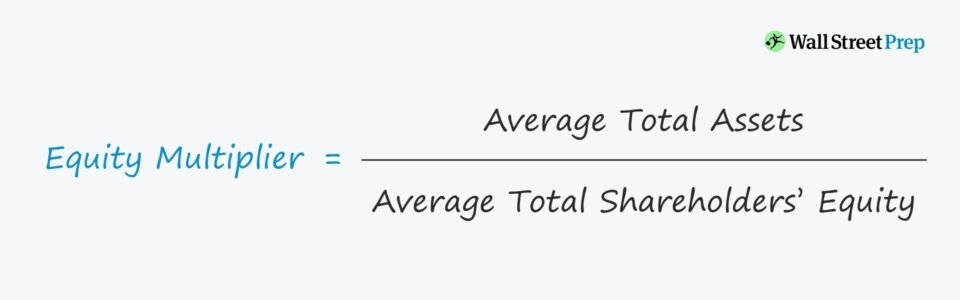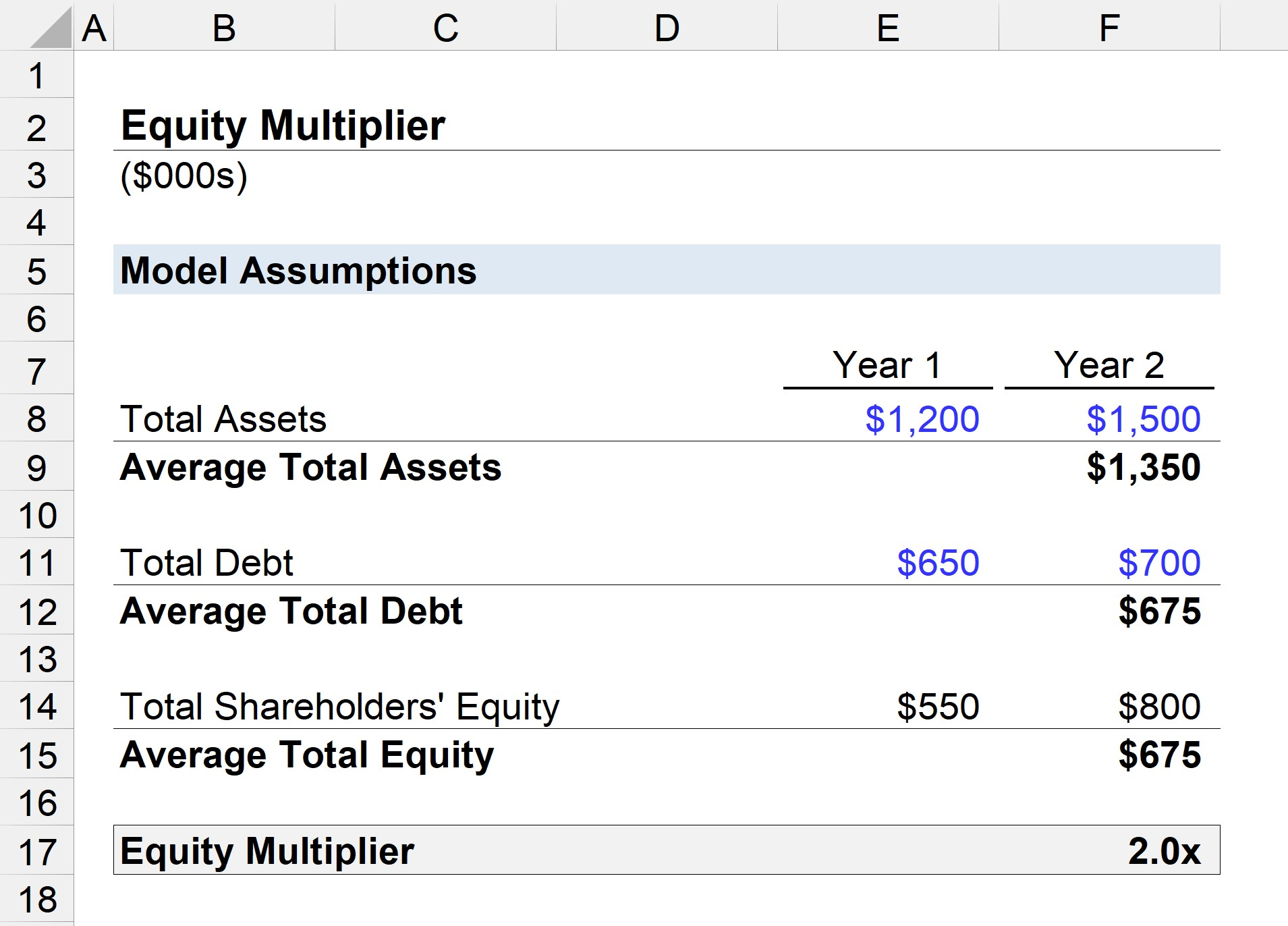Welcome to Wall Street Prep! Use code at checkout for 15% off.# Equity Multiplier

Guide to Understanding the Equity Multiplier## How to Calculate Equity Multiplier?

The formula for calculating the equity multiplier consists of dividing a company’s total asset balance by its total shareholders’ equity.

Equity Multiplier = Average Total Assets ÷ Average Total Shareholders’ Equity
• Average Total Assets = (Ending Total Assets + Beginning Total Assets) ÷ 2
• Average Total Shareholders’ Equity = (Ending Total Equity + Beginning Total Equity) ÷ 2

For instance, if a company has an equity multiplier of 2x, the takeaway is that financing is split equally between equity and debt.

## Equity Multiplier Formula

The equity multiplier is one of the ratios that make up the DuPont analysis, which is a framework to calculate the return on equity (ROE) of companies.

In the three-step DuPont analysis variation, the equity multiplier is multiplied by the net profit margin and asset turnover.

DuPont Analysis = Net Profit Margin × Asset Turnover × Equity Multiplier

Where:

• Net Profit Margin = Net Income ÷ Revenue
• Asset Turnover = Revenue ÷ Average Total Assets
• Equity Multiplier = Average Total Assets ÷ Average Shareholders’ Equity

Revenue and net income each represent income statement metrics, meaning that they measure across a period of time – whereas assets and equity are balance sheet metrics, which are the carrying values at a specific point in time.

To match the timing between the denominator and numerator among all three ratios, the average balance is used (i.e. between the beginning and end of period value for balance sheet metrics).

## What is a Good Equity Multiplier?

Higher equity multipliers typically signify that the company is utilizing a high percentage of debt in its capital structure to finance working capital needs and asset purchases.

More reliance on debt financing results in higher credit risk – all else being equal.

By contrast, a lower multiplier means that the company has less reliance on debt (and reduced default risk).

Therefore, a lower multiplier is usually perceived as better, since the company is relying more on equity contributed by the owners (e.g. founders, institutional investors), as well as its retained earnings.

• Low Multiplier: If the multiplier is “low”, the company either cannot obtain debt from lenders, or the use of debt is intentionally avoided by management – so continued operations are a positive signal that the current equity capital on-hand and retained earnings are sufficient.
• High Multiplier: If the multiplier is “high,” the company’s operations and asset purchases are financed primarily by debt, making it prone to default risk.

But as is the case for practically all financial metrics, the determination of whether a company’s equity multiplier is high (or low) is dependent on the industry average and that of comparable peers.

Another exception is for mature, established companies with high debt capacities, as one “economic moat” of the company is its access to financing with favorable lending terms (and ability to purchase inventory from suppliers at lower prices due to buying power).

## Equity Multiplier Calculator

We’ll now move to a modeling exercise, which you can access by filling out the form below.#### Get the Excel Template!Submitting...

## Equity Multiplier Calculation Example

For our illustrative scenario, we will calculate the equity multiplier of a company with the following balance sheet data.

Year 1 Financials:

• Total Assets = \$1.2m
• Total Debt = \$650k
• Total Shareholders’ Equity = \$550k

Year 2 Financials:

• Total Assets = \$1.5m
• Total Debt = \$700k
• Total Shareholders’ Equity = \$800k

To calculate the shareholders’ equity account, our model assumes that the only liabilities are the total debt, so the equity is equal to total assets subtracted by total debt.

Given these assumptions, we can calculate the average balance for each:

• Average Total Assets = \$1.35m
• Average Total Debt = \$675k
• Average Total Equity = \$675k

In the final step, we will input these figures into our formula from earlier, which divides the average total assets by the total shareholder’s equity.

• Equity Multiplier = \$1.35m Assets ÷ \$675k Equity = 2.0x

The company in our illustrative example has an equity multiplier of 2.0x, so the \$1.35m assets on its balance sheet were funded equally between debt and equity, with each contributing \$675k.Step-by-Step Online Course

### Everything You Need To Master Financial Modeling

Enroll in The Premium Package: Learn Financial Statement Modeling, DCF, M&A, LBO and Comps. The same training program used at top investment banks.